Anúncio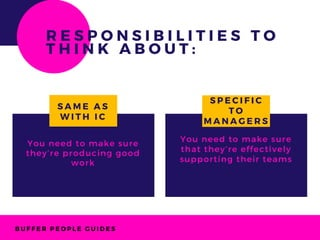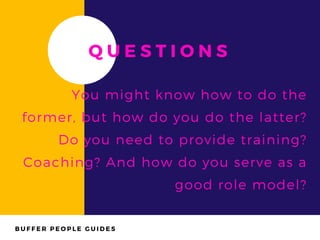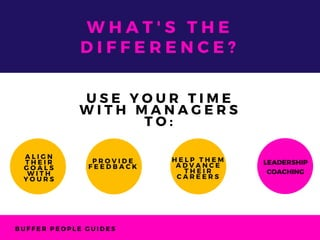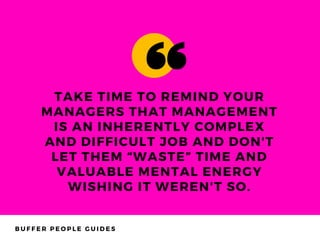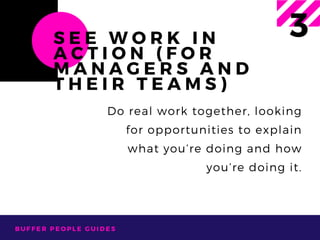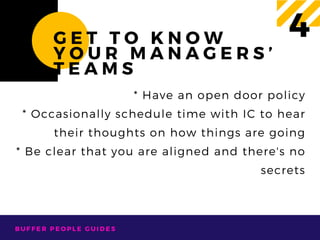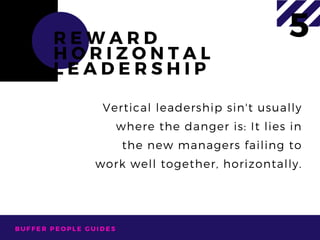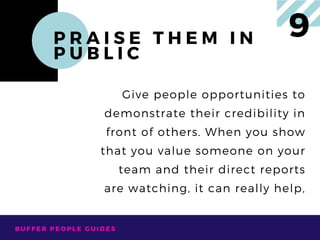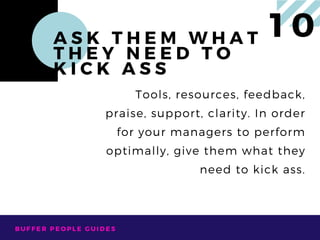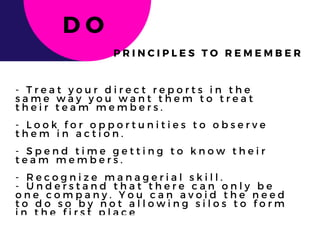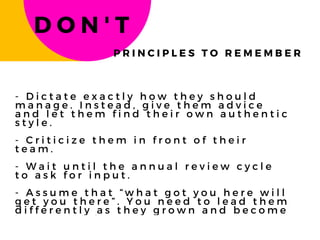1 de 20
Anúncio

### In the workplace

1. M A N A G E R S W O R K I N G B E T T E R W I T H BUFFERPEOPLEGUIDES
2. B U F F E R P E O P L E G U I D E S You need to make sure that they’re effectively supporting their teams You need to make sure they’re producing good work S A M E A S W I T H I C S P E C I F I C T O M A N A G E R S R E S P O N S I B I L I T I E S T O T H I N K A B O U T :
3. B U F F E R P E O P L E G U I D E S Q U E S T I O N S You might know how to do the former, but how do you do the latter? Do you need to provide training? Coaching? And how do you serve as a good role model?
4. B U F F E R P E O P L E G U I D E S 1 0 K E Y S T O   H E L P Y O U R D I R E C T R E P O R T S B E G R E A T M A N A G E R S
5. B U F F E R P E O P L E G U I D E S C H A N G E T H E F O C U S O F Y O U R C O A C H I N G 1 With individual contributors, you’d spend your time together digging into the details of specific projects. But with managers, you’ll dig into the details of their management.
6. B U F F E R P E O P L E G U I D E S W H A T ' S T H E D I F F E R E N C E ? A L I G N T H E I R G O A L S W I T H Y O U R S P R O V I D E F E E D B A C K U S E Y O U R T I M E W I T H M A N A G E R S T O : H E L P T H E M A D V A N C E T H E I R C A R E E R S LEADERSHIP COACHING
7. B U F F E R P E O P L E G U I D E S K N O W T H A T Y O U ’ L L T E A C H B Y M O D E L L I N G 2 Be sure that you are managing your people in the way that you expect them to manage their own teams.
8. B U F F E R P E O P L E G U I D E S TAKE TIME TO REMIND YOUR MANAGERS THAT MANAGEMENT IS AN INHERENTLY COMPLEX AND DIFFICULT JOB AND DON'T LET THEM “WASTE” TIME AND VALUABLE MENTAL ENERGY WISHING IT WEREN'T SO.
9. B U F F E R P E O P L E G U I D E S S E E W O R K I N A C T I O N ( F O R M A N A G E R S A N D T H E I R T E A M S ) 3 Do real work together, looking for opportunities to explain what you’re doing and how you’re doing it.
10. B U F F E R P E O P L E G U I D E S G E T T O K N O W Y O U R M A N A G E R S ’ T E A M S 4 * Have an open door policy * Occasionally schedule time with IC to hear their thoughts on how things are going * Be clear that you are aligned and there's no secrets
11. B U F F E R P E O P L E G U I D E S R E W A R D H O R I Z O N T A L L E A D E R S H I P 5 Vertical leadership sin't usually where the danger is: It lies in the new managers failing to work well together, horizontally.
12. B U F F E R P E O P L E G U I D E S T R E A T T H E M L I K E H I G H P O T E N T I A L T E A M M E M B E R S A N D C H A L L E N G E T H E M 6 High potentials, more than anyone, need to feel continually challenged. They need to understand the strategy or approach then be given the ball – to do what they do best.
13. B U F F E R P E O P L E G U I D E S G I V E T H E M E X P O S U R E 7 You should allow your managers to can gain valuable business knowledge and perspective. You should also make sure to give them credit for their work.
14. B U F F E R P E O P L E G U I D E S G I V E T H E M S P A C E 8  It means being a coach rather than a referee. A good coach chooses the right players, assesses their strengths and weaknesses and places each person in the position where they’ll perform their best.
15. B U F F E R P E O P L E G U I D E S P R A I S E T H E M I N P U B L I C 9 Give people opportunities to demonstrate their credibility in front of others. When you show that you value someone on your team and their direct reports are watching, it can really help,
16. B U F F E R P E O P L E G U I D E S A S K T H E M W H A T T H E Y N E E D T O K I C K A S S 1 0 Tools, resources, feedback, praise, support, clarity. In order for your managers to perform optimally, give them what they need to kick ass.
17. B U F F E R P E O P L E G U I D E S - T r e a t y o u r d i r e c t r e p o r t s i n t h e s a m e w a y y o u w a n t t h e m t o t r e a t t h e i r t e a m m e m b e r s . - L o o k f o r o p p o r t u n i t i e s t o o b s e r v e t h e m i n a c t i o n . - S p e n d t i m e g e t t i n g t o k n o w t h e i r t e a m m e m b e r s . - R e c o g n i z e m a n a g e r i a l s k i l l .   - U n d e r s t a n d t h a t t h e r e c a n o n l y b e o n e c o m p a n y . Y o u c a n a v o i d t h e n e e d t o d o s o b y n o t a l l o w i n g s i l o s t o f o r m i n t h e f i r s t p l a c e D O P R I N C I P L E S T O R E M E M B E R
18. B U F F E R P E O P L E G U I D E S - D i c t a t e e x a c t l y h o w t h e y s h o u l d m a n a g e . I n s t e a d , g i v e t h e m a d v i c e a n d l e t t h e m f i n d t h e i r o w n a u t h e n t i c s t y l e . - C r i t i c i z e t h e m i n f r o n t o f t h e i r t e a m .   - W a i t u n t i l t h e a n n u a l r e v i e w c y c l e t o a s k f o r i n p u t .   - A s s u m e t h a t “ w h a t g o t y o u h e r e w i l l g e t y o u t h e r e ” . Y o u n e e d t o l e a d t h e m d i f f e r e n t l y a s t h e y g r o w n a n d b e c o m e m o r e c o m p l e x . D O N ' T P R I N C I P L E S T O R E M E M B E R
19. B U F F E R P E O P L E G U I D E S T H A N K S ! : )
20. B U F F E R P E O P L E G U I D E S C O N T A C T D E B O R A H @ B U F F E R A P P . C O M A N Y C O M M E N T O R Q U E S T I O N ?
Anúncio